Author: Oscar Cronquist Article last updated on October 15, 2018

The array formula below counts how many times multiple text strings exist in a cell range. It is easy to add more text strings, adjust cell range D1:E1 horizontally. The formula takes into account if a text string exists multiple times in a single cell, see picture below.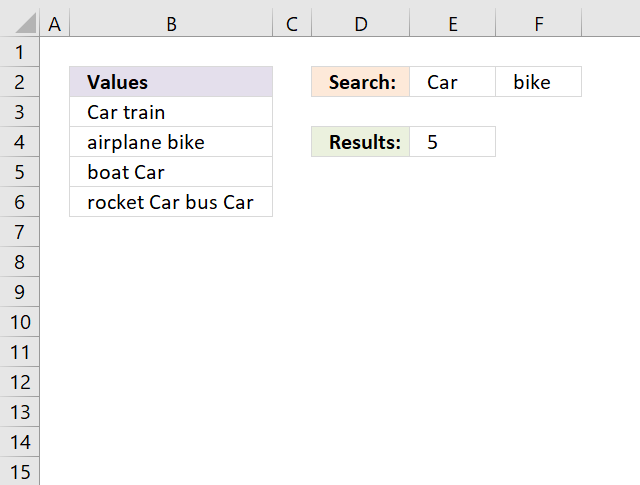Text strings "Car" and "bike" exist 5 times in cell range B3:B6.

### Case sensitive counting

Array formula in cell E4:

=SUM((LEN(B3:B6)-LEN(SUBSTITUTE(B3:B6,E2:F2,"")))/LEN(E2:F2))

or use this slightly larger regular formula:

=SUM(INDEX((LEN(B3:B6)-LEN(SUBSTITUTE(B3:B6,E2:F2,"")))/LEN(E2:F2),0,0))

### Case insensitive counting

Array formula in cell D2:

=SUM((LEN(B3:B6)-LEN(SUBSTITUTE(UPPER(B3:B6),UPPER(E2:F2),"")))/LEN(E2:F2))

or use this slightly larger regular formula:

=SUM(INDEX((LEN(B3:B6)-LEN(SUBSTITUTE(UPPER(B3:B6),UPPER(E2:F2),"")))/LEN(E2:F2),0,0))

### How to enter an array formula

1.  Select cell D2
2. Click in formula bar3. Paste above array formula
4. Press and hold CTRL + SHIFT
5. Press Enter

The formula bar displays curly brackets:

{=SUM((LEN(A2:A5)-LEN(SUBSTITUTE(A2:A5,D1:E1,"")))/LEN(D1:E1))}

### Explaining array formula in cell D2

Step 1 - Count characters in each cell

Len(A2:A5)

becomes

Len({"Car train"; "airplane bike"; "boat Car"; "rocket Car bus Car"})

and returns {9; 13; 8; 18}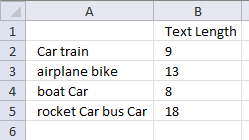Step 2 - Replace existing text with new text string

SUBSTITUTE(A2:A5,D1:E1,"")

becomes

SUBSTITUTE({"Car train"; "airplane bike"; "boat Car"; "rocket Car bus Car"},{"Car", "bike"},"")

and returns {" train", "Car train"; "airplane bike", "airplane "; "boat ", "boat Car"; "rocket  bus ", "rocket Car bus Car"}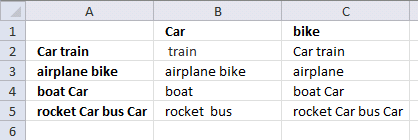Step 3 - Count characters in each value

LEN(SUBSTITUTE(A2:A5, D1:E1, ""))

becomes

LEN({" train", "Car train"; "airplane bike", "airplane "; "boat ", "boat Car"; "rocket  bus ", "rocket Car bus Car"})

and returns {6,9;13,9;5,8;12,18}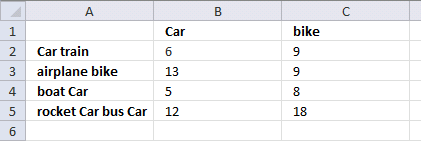Step 4 - Subtract

LEN(A2:A5)-LEN(SUBSTITUTE(A2:A5,D1:E1,""))

becomes

{9; 13; 8; 18} - {6,9;13,9;5,8;12,18}

an returns

{3,0;0,4;3,0;6,0}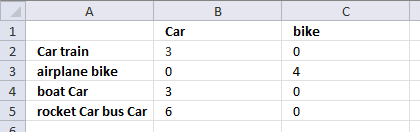Step 5 - Divide with length of each search string

(LEN(A2:A5)-LEN(SUBSTITUTE(A2:A5,D1:E1,"")))/LEN(D1:E1)

becomes

{3,0;0,4;3,0;6,0}/LEN(D1:E1)

becomes

{3,0;0,4;3,0;6,0}/{3,4}

and returns {1,0;0,1;1,0;2,0}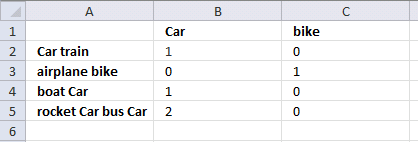Step 6 - Sum all values

SUM((LEN(A2:A5)-LEN(SUBSTITUTE(A2:A5,D1:E1,"")))/LEN(D1:E1))

becomes

SUM({1,0;0,1;1,0;2,0})

and returns 5 in cell D2

### Download excel *.xlsx file

Count multiple text strings in a cell rangev2.xlsx